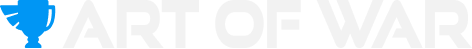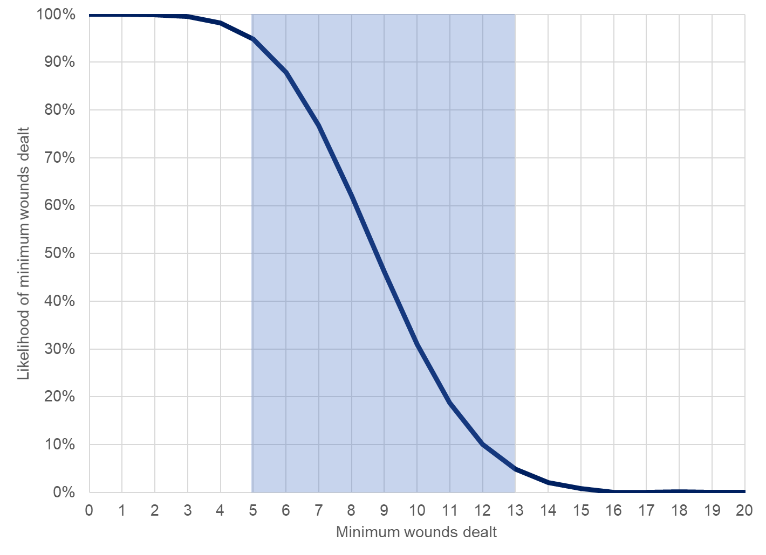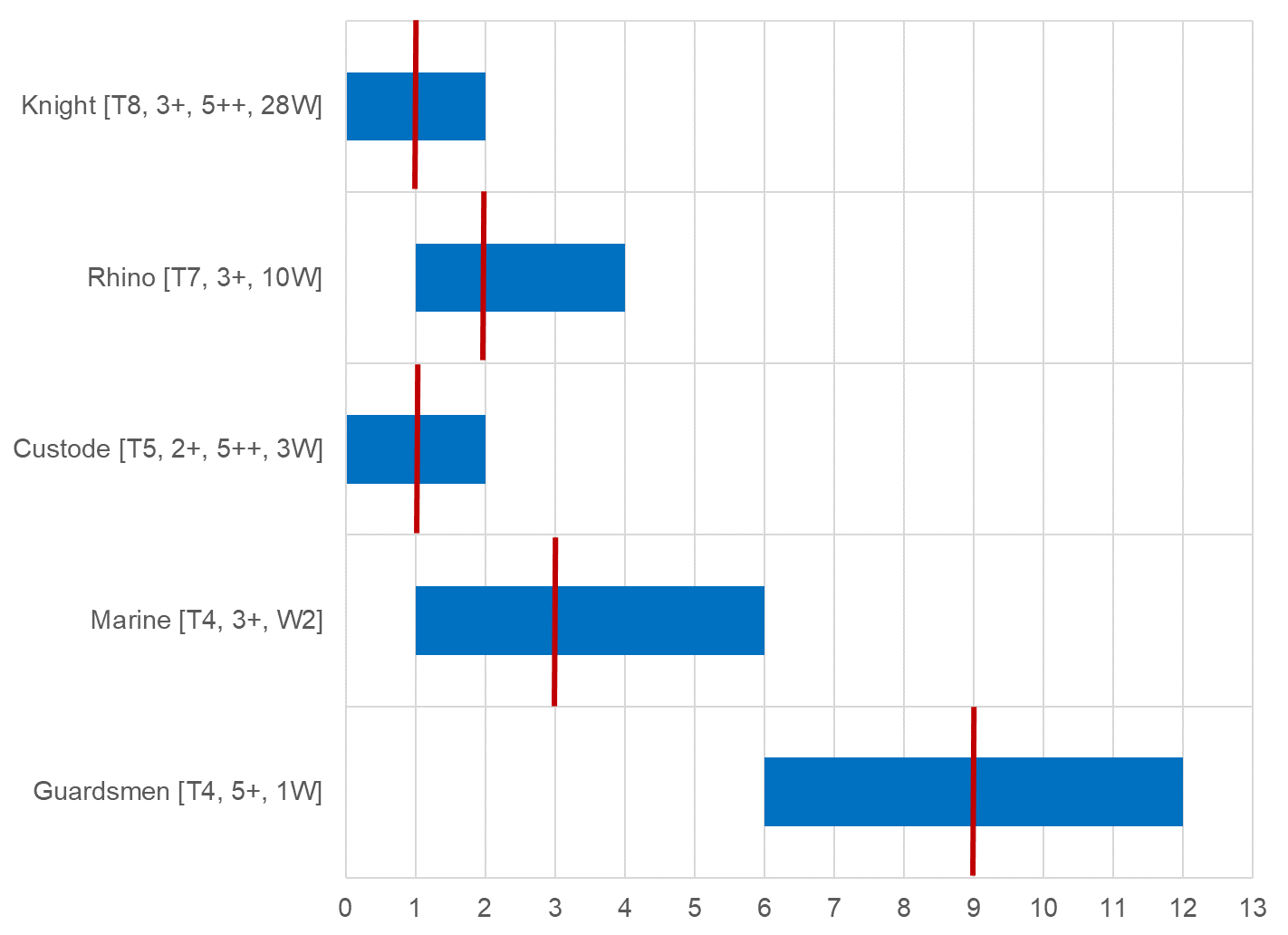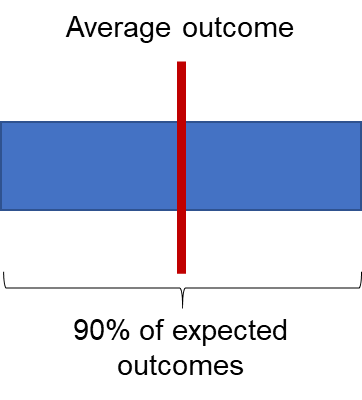# Mathammer 101The Art (and Science) of War: Introduction and preview of what is to come!

This week we welcome Adam Lane, one of the Art of War coaches and an absolute math wizard! He’s here to explain how to best use math with your favorite wargame.

The purpose of this is article is to provide you with an introduction to the concept of Mathhammer and give you with some quick practical tips to help you make better decisions at the tabletop. This is going to be one of a series of articles that we will be building on over time and is intended as a reference point / reminder for anyone who wants to know more about the analytical side of the game.

When you are at the table and engage in either a shooting or melee attack you are triggering a complex statistical process. You might be interested to know the type of analysis we were considering started in the 17th century as a result of an argument between two French gamblers who also happened to be mathematicians. In a very real sense, the analysis of random games led to the development of a unique branch of mathematics which we can now use to help us better understand 40k.

As I am sure you have experienced the basic attack sequence in 40K can generate many potential outcomes. As a competitive player it is important that you understand these outcomes so you can make better decisions. For example, if you throw a single hit roll, a single wound roll and there is a single save roll then there are 216 potential unique dice combinations of 1s, 2s, 3s, 4s, 5s and 6s. Of course, this is before we allow for re-rolls and rolls to modify damage which takes the number of potential outcomes comfortably into the tens of thousands.

To get a proper grip of how effective an attack is you would need to play a lot of games.  An alternative is to calculate the effectiveness of an attack which will give you a short cut to understanding the capability of your units.  Let’s start by looking at the structure of a single attack which can be visualised as follows:

The amount of damage an attack can produce is the total number of attacks multiplied by the total damage of each attack. This defines the maximum damage potential from any given attack. In contrast the hit, wound and the save rolls act to reduce the potential damage output of a given attack. Therefore, we think it is helpful to consider these components separately.

In theory it is possible to achieve the maximum damage output, but it is also unlikely to happen (sometimes very unlikely to happen).  There is also a range of outcomes between these two extremes which in statistics is known as the distribution of outcomes.  If we can calculate this distribution, then we will know how likely it is to get a certain outcome and will be better positioned to make calculated decisions.  A player who can accurately calculate this distribution would never again waste resources by overcommitting or under committing attacks to an enemy unit.  Given the fine margins in competitive games my aim to is to help you get a better understanding of this process to give you an edge which will then hopefully translate into more wins.

Often you will hear gamers complain their dice roll was “less than average” and hence they have been unlucky. I want you to know is that an average typically means that half the time you will do worse than the average and half the time you will do better.  In other words, you shouldn’t “expect” to get the average outcome in the same way you wouldn’t always expect to get a 4+ when you roll a single die.

Having said the above, calculating the average outcome is a very useful place to start.  We share a quick 3-step process for calculating averages.  We demonstrate this by looking at an example: 10 Space Marine Intercessors shooting their Auto Bolt Rifles into a unit of 30 Ork Boyz.

This ready reckoner is quick enough that you will be able to apply this technique in game and with a bit of practice it will become second nature. I suspect many of you already do this regularly – in which case feel free to skip the section – if not then please read on!

Step 1:  Calculate the total potential damage

To complete this step, multiply the total number of attacks by the total damage characteristic of the weapon.

Each of the 10 Intercessors carries an Auto Bolt Rifle and they provide 3 attacks each so the total number of attacks is 10 x 3 = 30.  The damage characteristic for this weapon is 1 so the total damage potential is 30 x 1 = 30.  This is illustrated below:

There are 30 Ork Boyz in total and each one has a single wound which means in theory the Space Marine unit could kill all 30 Ork Boyz in a single round of shooting.  The next step will help us estimate what is likely to happen in practice.

Step 2: Calculate the effectiveness of the attack.

For each of the hit, wound and save rolls we calculate the number of faces of each die that would lead to a successful outcome for the attack:

• In this case the Intercessors hit on a 3+ which means the number of potential successes on a single die is 4 (i.e. a roll of 3, 4, 5 or 6).
• The strength of the attack and the toughness of the Orks are both 4 so the Space Marines will wound on a 4+ which means the number of potential successes on a single die is 3 (i.e. a roll of 4, 5 or 6)
• The Ork player gets a 6+ save against this attack which means the number of potential successes on a single die is 5 (i.e. a roll of 1, 2, 3, 4 or 5)
• Multiplying these numbers together we get 4 x 3 x 5 = 60.

We can use this number to roughly calculate the average number of successful attacks.  To do this just half the number that you just calculated and stick a % sign on the end:

• Half of 60 = ~30% (e.g. roughly 9 out of 30 attacks will be successful)

A visual representation of why this shortcut works is shown below:

Our approximation gives us an average number of successful wounds of 30% while the true answer is 27.7%.

Step 3:  Calculate the expected damage output

As a final step just multiply the total damage potential calculated in step 1 by the attack efficiency calculated in step 2.  The calculation is shown below:

So the Space Marine player who shoots 10 Intercessors into a squad of 30 Ork Boyz will kill 9 Orks on average.  Remember the right way to think about this is that 50% of the time the Space Marine player will do better than this and 50% of the time the player will do worse than this estimate.

The reason why we suggest this approximation is that it should be easier to do when you are starting out and under time pressure at the table.  With a bit of practice, you will be able to do the full calculation in your head but this shorthand will likely be enough to get you going and still give you a good gauge of the average damage output of your units.

This was intended as a simple example and we will build on this in future articles to show how to incorporate the more complex interactions  For example, we will show you how to quickly allow for re-rolls, modifiers and feel no pain mechanics.

Extending the basic example

Let’s extended the analysis of the Space Marine unit shooting at the Ork Boyz and calculate the full distribution of potential outcomes (this is shown in the chart below).   This calculation is a lot more involved and is difficult to replicate at the table top.

The vertical axis shows the likelihood of generating a minimum number of wounds while the horizontal axis states the minimum number of wounds generated for a given level of success.This analysis shows that if you were to make 100 attacks then you would expect to kill between 5 and 13 Ork Boyz 90% of the time (this is shown by the shaded blue area).

Using this type of analysis can give you a very good gauge about what might happen most of the time.  For example, you should almost never expect to kill more than 18 Ork Boyz in a single round of shooting (if you are interested this should only ever happen once in 4,545 attacks).  You may not trust the maths so try it out using 30 dice and let me know how long it takes to get 18 successful attacks.

Finally, we wanted to show you a preview showing how the efficiency of the Auto Bolt rifle attack sequence is when applied to a range of different target:The intention here is analyse the efficiency of the attack against a wide range of different unit types so you can help design a well-rounded list.  This can help you answer whether you have enough anti-horde firepower or enough anti-tank. This type of work is probably best reserved for the army design phase and for creating crib sheets for your more important damage dealing units.

Final thoughts

This article was intended as quick introduction to Mathhammer and provide you with some helpful hints and tips.  We will build on this type of analysis in future articles and if you have any specific questions or ideas then please do let us know.

If you want to catch more of Adam, check out his Mathhammer clinics in the Art of War War Room!